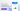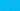# What Is Cross Elasticity of Demand?

Would a hike in gasoline price increase the demand for ride sharing and public transport services? Would an increase in the price of dairy products affect the demand of alternatives like soy milk? These are questions the economic principle of cross elasticity of demand seeks to answer. It measures the demand for a particular product when the price of another product changes. Keep reading to know more about cross elasticity of demand and how it works

## Cross Elasticity of Demand Explained

Generally, demand for one product depends on the price of other products, which may be complementary or substitutes. Cross elasticity of demand helps to determine the effect of the price of these other products. It evaluates the relationship between two products when the price of one of them changes. It does this by measuring the increase or decrease in the demand for a product following the change in the price of another product.

For example, an increase in the price of Coke might increase the demand for Pepsi. Similarly, a decrease in the price of Coke might decrease the demand for Pepsi. Now, cross elasticity of demand(XED) measures the degree of impact in which the changes in Coke’s price have on the changes in the demand for Pepsi.

## How to Calculate the Cross Elasticity of Demand

We calculate cross elasticity of demand by dividing the change in the percentage of the demand for a specific good by the change in percentage in the price of another product. As such, the cross elasticity of demand formula is expressed as follows:

XED = % Change in QD ÷ % Change in Price

where:

XED = Cross Elasticity of Demand

QD = Quantity Demanded

## How Cross Elasticity of Demand Works

In ascertaining the demand for a product, the cross elasticity of demand formula produces two results, i.e, the product is categorized as a complement or a substitute. However, this depends on the value realised following the calculation, which may be positive or negative.

Where positive, the good is regarded as a substitute. This means that the goods are direct competitors, in which case an increase in the price of one will lead people to opt for the other.

For instance, in the example above, there is no doubt that the increase in Coke’s price will cause an increase in the demand for Pepsi. This is because individuals that can no longer afford Coke will opt for Pepsi, an alternative product. As such, a calculation using the cross elasticity of demand formula will yield a positive result establishing that the goods are substitutes.

On the other hand, where the cross elasticity of demand value is negative, it establishes that the goods are complementary. What this means is, an increase in the price of one good will result in lower demand for the other product. That other product is therefore a complementary product which depends on the demand for the primary product to thrive. Going by the law of demand, an increase in primary product will result in lower demand, which also affects demand for its complementary goods.

## The Relevance of Cross Price Elasticity

Cross elasticity of demand allows businesses to understand the market better. In turn, it allows them to determine the price to be attached to their products.

For instance, products without substitutes can be priced higher. On the other hand, complementary products can be priced based on the relationship with other relevant products, as established by the result of the cross elasticity of demand calculation results

## We Can Help

GoCardless helps you automate payment collection, cutting down on the amount of admin your team needs to deal with when chasing invoices. Find out how GoCardless can help you with ad hoc payments or recurring payments.